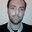Question-and-Answer Resource for the Building Energy Modeling Community
Get s tarted with the Help page

# Output file - electricity tariffHi! I modeled my buiding with OpenStudio. I'm also using jeplus to change the HVAC setpoints during the night(at night electricity costs changes) so I can find the setpoints that reflects the lower electricity costs. Changing my HVAC setpoints during the night will give me thousands of simulations. I'm trying to find in my *.rdd file any output variable that is related with tariffs/costs but with no success. As changing HVAC setpoints during the night will give me thousands of simulation jobs it's impossible to add the electricity tariff to an excel spreadsheet(after using ReadVarsEso) by hand and then multiply each hour tariff by the each hour Facility Total Purchased Electric Power and then obtain the Facility Total Purchased Electric cost for the run period(1 week). Exists any measure that can add the tariff to the *.eso file and then multiply the tariff by the Facility Total Purchased Electric Power? Do you know any other way I can solve this problem? Thank you

edit retag close merge delete

Sort by » oldest newest most voted

There is no output variable for each timestep related to the calculation of tariffs. You can enter costs that change for each hour using the Real Time Pricing Charge Schedule Name field of the UtilityCost:Tariff object in EnergyPlus. From that the total energy cost for what ever time period you simulate will be calculated. Could you clarify why you need the output of the cost each hour?

You might be able to use EMS to do some calculation for each timestep if you really need the output for each hour.

If you are interested in adding this capability, you can let the development team know using uservoice:

https://energyplus.uservoice.com/foru...

more

Hi JasonGlazer!Thank you for your answer.I will try to explain better my problem. I have thousands of simulations to perform and I'm using jeplus to realize my simulations. In jeplus we have to define in the *.rvi file the output variables that we want based on the *.rdd. So I need one output variable that can give me the total cost for the run period(1week). The cost output for each timestep is not important. My idea is to run in jeplus a run period simulation with all variables set to "run period".

So I can go to "SimResults.csv" provided by jeplus to see which job as the lowest electricity consumption. Then I can set the variables output to hourly and perform another simulation in jeplus so I can go to the job with the lowest electricity consumptions to pick up the correspondent setpoints for each hour of the run period. My main doubt is how to perform huge amount of simulations "automatically" and see at the same time the electricity costs for the run period. Ther is another way I can do this? Thank you

I think you can get to the normal tabular outputs using jeplus and the tabular outputs include the tariff results. Look at the

http://www.jeplus.org/wiki/doku.php?i...

See the section on "Read from E+ CSV tabular report"